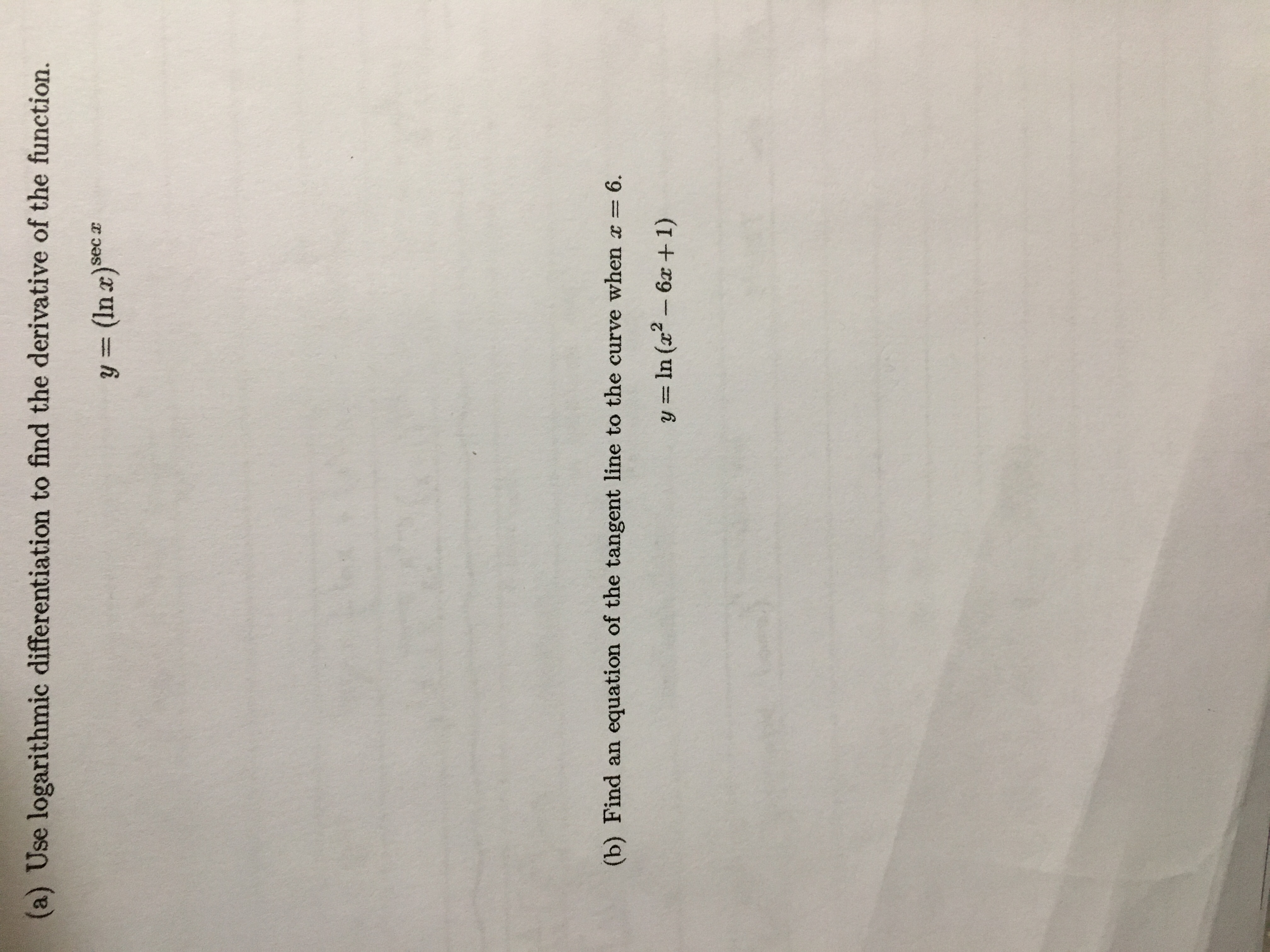# (a) Use logarithmic differentiation to find the derivative of the function.y (In x)seca(b) Find an equation of the tangent line to the curve when x 6.y = ln (x2 -6x+1)

Question
12 viewshelp_outlineImage Transcriptionclose(a) Use logarithmic differentiation to find the derivative of the function. y (In x)seca (b) Find an equation of the tangent line to the curve when x 6. y = ln (x2 -6x+1) fullscreen
check_circle

Step 1

We’ll answer the first question since the exact one wasn’t specified. Please submit a new question specifying the one you’d like answered.

Step 2

To determine the derivative of given function.

Step 3

Given function:

...

### Want to see the full answer?

See Solution

#### Want to see this answer and more?

Solutions are written by subject experts who are available 24/7. Questions are typically answered within 1 hour.*

See Solution
*Response times may vary by subject and question.
Tagged in

### Calculus